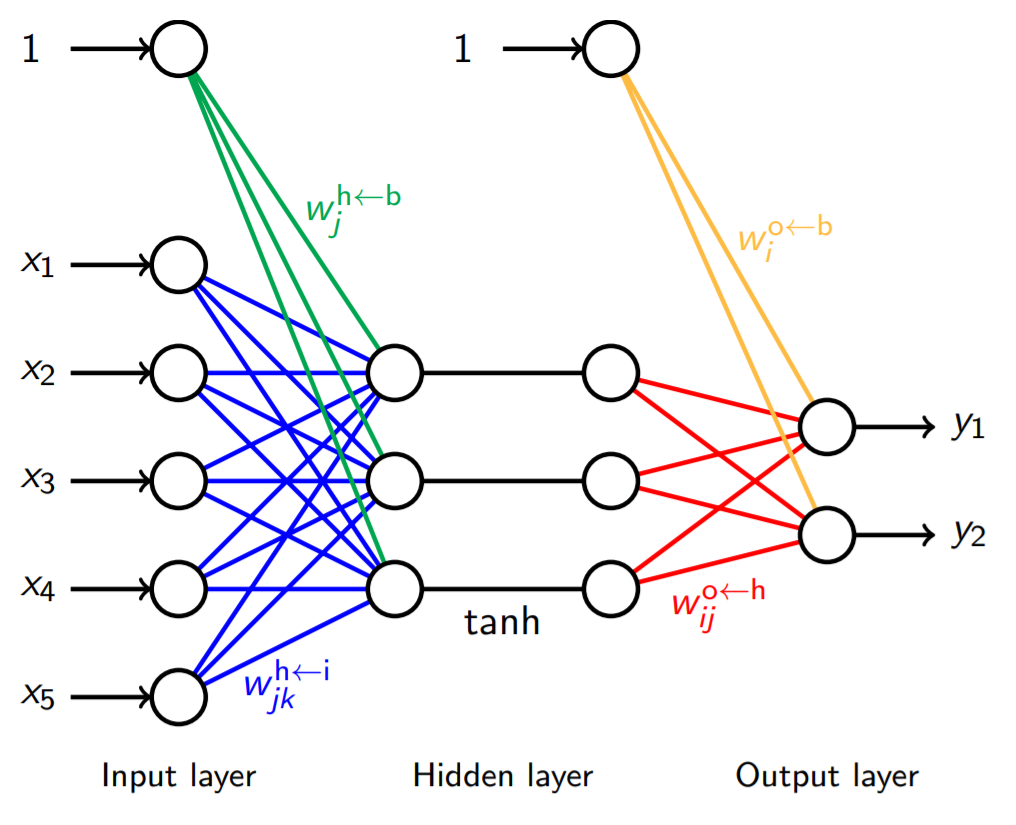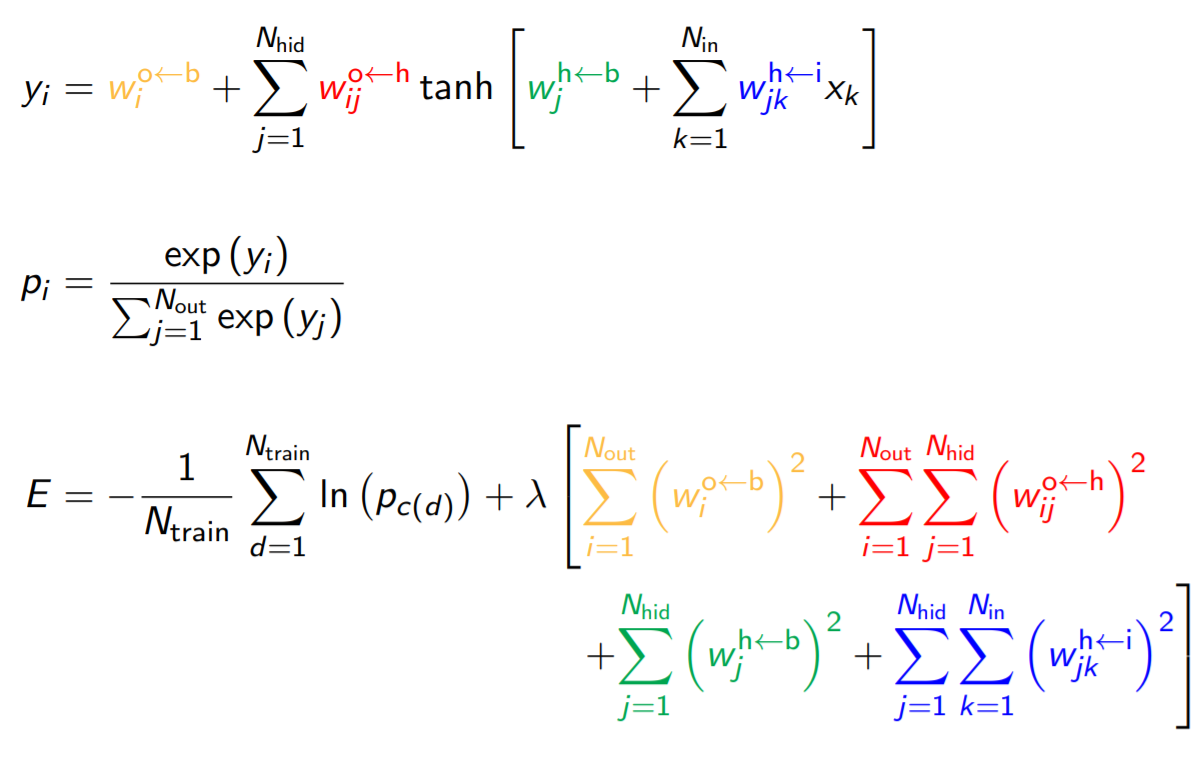# Yusuf Hamied Department of ChemistryNeural networks are nothing but a complicated best-fit problem, similar in some ways to simple linear regression (fitting the function $y=mx+c$ to some data).  To find a neural network's fits to a dataset, one needs to minimise its "loss" or "cost" function, $E$, which is a function of all the parameters in the network.  Such cost functions define an ($N_w+1$)-dimensional landscape in parameter space, where $N_w$ is the number of parameters in the model.  This landscape has minima, which are the locations of "best-fit" solutions to the given dataset, which we would like to find.

This problem is analogous to the problem of finding stable atomic arrangements: $N$ atoms may be placed at positions in 3D space, and there is an associated potential energy, $V$, for a given configuration.  The stable configurations are those that give minima in $V$, so the atomic positions must be varied to search for such minima.  The atomic positions are analogous to the neural network weights, and $V$ is analogous to the cost function, $E$.

My research uses GMIN, OPTIM and PATHSAMPLE (Wales group software) to explore the landscapes defined by neural network cost functions and find different local minima corresponding to different neural network solutions.  The datasets used to train and test these neural networks are from Intensive Care Units (ICUs), which have a very large volume of data.  I am looking for ways to improve predictions made by neural network models by using this potential energy landscape approach.

## Publications

In-situ uniaxial drawing of poly-L-lactic acid (PLLA): Following the crystalline morphology development using time-resolved SAXS/WAXS
EL Heeley, K Billimoria, N Parsons, Ł Figiel, EM Keating, CT Cafolla, EM Crabb, DJ Hughes
– Polymer
(2020)
193,
122353
Stress-oscillation behaviour of semi-crystalline polymers: the case of poly(butylene succinate)
C Wan, EL Heeley, Y Zhou, S Wang, CT Cafolla, EM Crabb, DJ Hughes
– Soft matter
(2018)
14,
9175

## Telephone number

01223 336530 (shared)